# Function (mathematics) facts for kids

Kids Encyclopedia Facts

In mathematics, a function is a mathematical object that produces an output, when given an input (which could be a number, a vector, or anything that can exist inside a set of things).

So a function is like a machine, that takes values of x and returns an output y. The set of all values that x can have is called the domain, and the set that contains every value that y can have is called the codomain. A function is often denoted by italic letters such as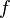$f$,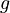$g$,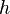$h$.

If this happens, then we say that y is a function of x, and we write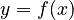$y=f(x)$. Here,$f$ is the name of the function, and one writes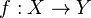$f:X \to Y$ (function from X to Y) to represent the three parts of the function: the domain (x), the codomain (y), and the pairing process (the arrow).

An example of a function is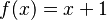$f(x)=x+1$. One gives a natural number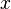$x$ (0,1,2,3...) as the input, and gets a natural number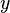$y$, which is$x$ +1 (1,2,3,4...) The idea of a function has been set up to cover all sorts of possibilities. The function doesn't have to be an equation. The main idea is that inputs and outputs are paired up somehow—even if the process might be very complicated.

## Metaphors

### Tables

The inputs and outputs can be put in a table like the picture; this is easy if there is not too much data.

### Graphs

In the picture, one can see that both 2 and 3 have been paired with c; this is not allowed in the other direction, as 2 could not output c and d at the same time (each input can only have one output). All of the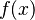$f(x)$ (c and d in the picture) are usually called the image set of$f$, and the image set can be the entire codomain or one of its subset. One can say that the image set of a subset A of the domain is f(A). If the inputs and outputs have an order, then it is easy to plot them on a graph: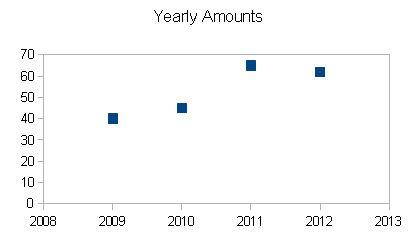In that way, the image comes on the image of the set A.

## History

In the 1690s, Gottfried Leibniz and Johann Bernoulli used the word "function" in letters between them, so the modern concept began at the same time as calculus.

In 1748, Leonhard Euler gave the following definition for function:

"A function of a variable quantity is an analytic expression composed in any way whatsoever of the variable quantity and numbers or constant quantities."

and then in 1755:

"If some quantities so depend on other quantities that if the latter are changed the former undergoes change, then the former quantities are called functions of the latter. This definition applies rather widely and includes all ways in which one quantity could be determined by other. If, therefore, x denotes a variable quantity, then all quantities which depend upon x in any way, or are determined by it, are called functions of x."

Usually, Peter Dirichlet is credited with the first modern definition of function (formulated in 1837). It is often used in schools until the second half of the 20th century:

"y is a function of a variable x, defined on the interval a < x < b, if to every value of the variable x in this interval there corresponds a definite value of the variable y. Also, it is irrelevant in what way this correspondence is established."

In 1939, the Bourbaki generalized the Dirichlet definition, and gave a set-theoretic version of the definition as a correspondence between inputs and outputs; this was used in schools from about 1960.

Finally in 1970, Bourbaki gave the modern definition as a triple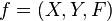$f = (X,Y,F)$, with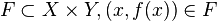$F\sub X\times Y, (x,f(x))\in F$ (i.e.$f:X \to Y$ and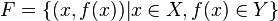$F=\{(x,f(x))|x\in X,f(x)\in Y\}$). X is called the domain of f, Y its codomain, and F its graph. The set of all elements of the form f(x), where x ranges over the elements of the domain X, is called the image of f. The image of a function is a subset of its codomain so it might not coincide with it.

## Types of functions

• Elementary functions - The functions that are usually studied in school: fractions, square roots, the sine, cosine and tangent functions and some other functions.
• Non-elementary functions - Most of them do not use operations that we don't learn in school (like + or -, or powers). Many integrals, for example, are non-elementary.
• Inverse functions - Functions that undo another function. For example: if F(x) is the inverse of f(x)=y, then F(y)=x. Not all functions have inverses.
• Special functions: Functions that have names. These include trigonometric functions such as sine, cosine and tangent. Functions like f(x)=3x (three times x) are not called special functions. Special functions can be elementary, non-elementary or inverses.

## Related pagesFunction (mathematics) Facts for Kids. Kiddle Encyclopedia.